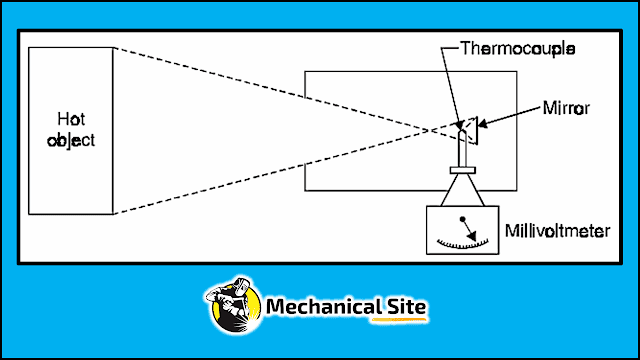Diagram of radiation pyrometer.

What is radiation pyrometer? A device which measures the total intensity of radiation emitted from a body is called radiation pyrometer.
The elements of a total radiation pyrometer are illustrated in the figure. It collects the radiation from an object (hot body) whose temperature is required. A mirror is used to focus this radiation on a thermocouple.

This energy which is concentrated on the thermocouple raises its temperature, and in turn, generates an e.m.f. This e.m.f. is then measured either by the galvanometer or potentiometer method. Thus the rise of temperature is a function of the amount of radiation emitted from the object.

• The temperatures of moving objects can be measured.
• Higher temperature measurement is possible than that possible by thermocouples etc.
• The average temperatures of the extended surface can be measured.
• The temperature of the objects which are not easily accessible can be measured.

#### What is the total radiation pyrometer?

The total radiation pyrometer receives all the radiation from a particular area of a hot body. The term total radiation includes both visible and invisible radiations. It consists of a radiation receiving element and a measuring device.

#### What does a pyranometer measure?

A pyranometer is a type of actinometer used for measuring solar irradiance on a planar surface and it is designed to measure the solar radiation flux density (W/m2) from the hemisphere above within a wavelength range of 0.3 μm to 3 μm.

#### What is the difference between a thermometer and a pyrometer?

The difference between a thermometer and a pyrometers is that a thermometer is an apparatus used to measure temperature while a pyrometer is a thermometer designed to measure high temperatures.
–>